Dimas Buntarto

# Introduction

Regression is one method to determine the causal relationship between variables with other variables. In simple regression analysis, the relationship between variables is linear, where changes in the variable X will be followed by changes in the variable permanently.

Regression is an analytical method commonly used to see the effect between two or many variables. Generally, regression analysis is used to make predictions or forecasts. Meanwhile, the relationship between these variables is functional which is realized in a mathematical model.

The variable whose value is predicted is called the target. In regression, the target has a continuous value.

In the real world, sometimes found targets that are not normally distributed. This sometimes affects the model’s performance on the data.

Before doing the analysis, sometimes the target is transformed first, so that the distribution is close to normal.

This paper will try to determine the effect of target transformation on the analysis using regression models. The data we will use is the abalone dataset.

# Objective

• Knowing the effect of target transformation on the regression method used to predict the age of abalone.

# Methods

1. Getting dataset from website http://archive.ics.uci.edu/ml/datasets/Abalone.
2. Checking the dataset.
3. Doing Exploratory Data Analysis to the dataset.
4. Doing feature enginering to the dataset.
5. Comparing regression models with and without target transformation.

The regression models that we will use are:
1. Linear Regression
2. K Nearest Neighbors
3. Decision Tree
4. Support Vector Machine

As for the target transformation, we will use the np.sqrt

he dataset is retrieved from http://archive.ics.uci.edu/ml/datasets/Abalone. Given is the at-tribute name, attribute type, the measurement unit and a brief description.

# Getting The Dataset

We get the dataset first. We get the abalone dataset through the site http://archive.ics.uci.edu/ml/datasets/Abalone

From the table above we can see that the data has 4,177 samples with 9 features.

# Checking The Dataset

We first check the condition of the data which includes the types of features and data descriptions.

All features are float and integer except for the `Sex` feature. From the information above, we can find out that there are no missing values in the data.

We look at the description of the data to ensure that the data is informed.

Information on the data states that there are no missing values in the data. However, if you look at the data description, there is a value of 0 in the height measurement (the `Height` column). This 0 value is probably a measurement error in the data so we have to deal with it first.

## Handling measurement error

The data in the `Height` column may have measurement errors. We first check the position and number of the 0 values.

There are 2 samples that are estimated to have measurement errors in the `Height` column. The two empty values are in the infant gender (I).

Since there are only 2 samples, we can omit the two values. It’s just that in this paper we will replace it with the average value for the sex of infant (I).

We can see that there are no empty values in the dataset.

# Splitting the Dataset

Before we separate the data into train data and test data, we will first determine the age of the abalone. The age of abalone is obtained by adding the number of rings by 1.5.

The age of abalone has been determined.

Next, we will separate the data into groups of train data and test data. We will determine the size of the test data as much as 30% of the dataset

To make it easier for the next step, we will separate the existing variables.

# Exploratory Data Analysis

## Sex feature

Let’s start analyzing data train. We start by looking at the gender condition (the `Sex` column). Because the type of data on gender is categorical data, we will analyze it using a pie chart.

If we look, the sex distribution is quite balanced, which is about 30 percent for each gender.

We will still use this categorical feature in later modeling.

We will use one-hot encoding for further processing of this categorical feature.

## Numerical features

Next, let’s look at the distribution of the numerical features.

From the graph above, we can see that the numerical features are not normally distributed, except for the `Height` feature which looks slightly normally distributed. The `Length` and `Diameter` features have values that are distributed to the right of the curve, which means that their skewness is negative. The other features have a value distribution that is on the left of the curve, which means the skewness is positive.

Let’s see if there is an outlier in this numeric features

We can see that every numerical feature has an outlier. The number of outliers is the possibility that the data is not normally distributed.

We will not remove this outlier because we fear that we will lose information in the dataset.

For numerical features, in the next process we will scale continuous features with `MinMaxScaler`

## Target distribution

Next, we will look at the target distribution (`Age` column)

In the graph above, we can see that the target is not normally distributed and the possible value distribution is on the left of the curve, which means that the skewness is positive.

To make sure, we check if there is an outlier in the target.

The existence of outliers that cause the target is not maximally distributed. We also will not remove these outliers for fear that we will lose information on the dataset.

We will perform transformations on the target in the hope of improving the performance of the model.

## Pearson correlation

We will see how the correlation between features, especially numerical features with target.

Each numerical feature has a linear correlation with the target, only in the range of 0.5 which means the linearity to the target is neither too strong nor weak (moderate).

Furthermore, we can see it in the following scatter graph

We can see that there is a linear relationship between the numerical features and the target, even if calculated by Pearson correlation is moderate.

# Preprocessing and Feature Engineering

We first perform categorical feature engineering. We will use one-hot-encoding to encode the categorical features.

We can see that there is an increase in the column in the dataset to 11. Let’s look at the train set for the result.

Next, we separate features with the target.

# Modelling

We will first scale the features with `MinMaxScaler`. We will also use a `pipeline` to simplify the process. We will also use `GridSearchCV` to help estimate the best parameters, where cv equals 5.

For the process of using transformed targets, we will use the `TransformedTargetRegressor`.

We will transform the target with `np.sqrt`.

## Linear regression

We start with a linear regression model. For linear regression, the parameter we estimate is `fit_intercept`.

Linear regression without target transformation

Linear regression with target transformation

## Nearest Neighbors Regression

The parameter we will estimate is `n_neighbors`

Nearest Neighbors regression without target transformation

Nearest Neighbors regression with target transformation

## Decision Tree regression

The parameters we will set for the Decision Tree regression are `max_depth` and `max_features`

Decision Tree without target transformation

Decision Tree with target transformation

## Support Vector Regression

The parameters we will be setting are `C` and `gamma`.

Support Vector Regression without target transformation

Support Vector Regression with target transformation

Calculation of all the models above, we summarize in the following table.

# Discussion

In linear regression, the target transformation is able to reduce the mean square error value. It is even able to improve the performance of the model. However, the target transformation in linear regression actually reduces the complexity of the model. This can be seen when using linear regression with a transformed target, the model actually experiences underfitting.

In KNN regression, the model has complexity to the data (overfitting) either without or with the target transformation. Transforming the target in this model actually reduces the model’s performance and increases the mean square error value. Although the target transformation is able to slightly cause the model to be more general in the use of the same model.

The effect of the target transformation is quite good shown in the Decision Tree regression. Target transformation is able to reduce the mean square error value. Although it reduces the complexity of the model, the target transformation is able to make this model more general to the data.

Target transformation has an unfavorable effect on the SVR model. The target transformation actually increases the mean square error value and also reduces the performance of the model. The target transformation in this model also increases the complexity of the model, so that the model becomes less general to the data.

# Conclusion

From all the calculations above, it can be concluded:

1. Target transformation has a different effect on each model.
2. Of the four models used above, the model that can be used as a predictor is linear regression without model transformation. Because the model is the most generalized to the data, even though it is not the one with the smallest mean square error.

# Further Analysis

In the future, it is necessary to analyze using other regression models as well as other target transformation methods, such as `boxcox`, `np.log1p`, `np.log` and so on.

# References

--

--

--

## More from Analytics Vidhya

Analytics Vidhya is a community of Analytics and Data Science professionals. We are building the next-gen data science ecosystem https://www.analyticsvidhya.com

## Testing Different Models for the Titanic Dataset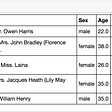## Object Tracking – MLT solution for the METI / NEDO Edge AI competition## Market Regime Determination with Machine Learning## Engineering behind RL## Building a *smarter* BeerBot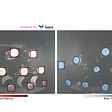## Introduction to Reinforcement Learning. Part 3: Q-Learning with Neural Networks, Algorithm DQN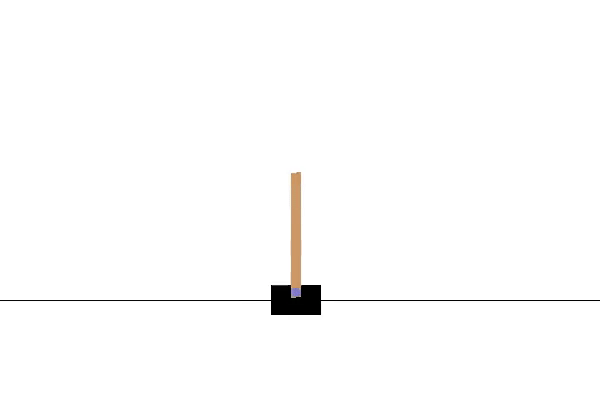## Transformer-XL Explained: Combining Transformers and RNNs into a State-of-the-art Language Model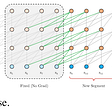Data Analyst

## Binary Classification Through Logistic Regression — Analytics Mag## Hypothesis, Parametric and Non-Parametric Testing## Uranium Price Prediction Using Multiple Linear Regression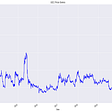## Understanding logistic regression